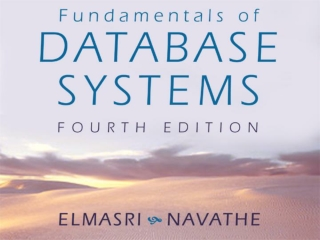DownloadDownload PresentationChapter 3

# Chapter 3

Télécharger la présentation## Chapter 3

- - - - - - - - - - - - - - - - - - - - - - - - - - - E N D - - - - - - - - - - - - - - - - - - - - - - - - - - -
##### Presentation Transcript

1. Chapter 3 Data Modeling Using the Entity-Relationship Model

2. FIGURE 3.1A simplified diagram to illustrate the main phases of database design.

3. FIGURE 3.2An ER schema diagram for the COMPANY database.

4. FIGURE 3.3Two entities, employee e1 and company c1, and their attributes.

5. FIGURE 3.4A hierarchy of composite attributes.

6. FIGURE 3.5A complex attribute: AddressPhone.

7. FIGURE 3.6Two entity types, EMPLOYEE and COMPANY, and some member entities of each.

8. FIGURE 3.7The CAR entity type with two key attributes, Registration and VehicleID.

9. FIGURE 3.9Some instances in the WORKS_FOR relationship set, which represents a relationship type WORKS_FOR between EMPLOYEE and DEPARTMENT.

10. FIGURE 3.10Some relationship instances in the SUPPLY ternary relationship set.

11. FIGURE 3.11A recursive relationship SUPERVISION between EMPLOYEE in the supervisor role (1) and EMPLOYEE in the subordinate role (2).

12. FIGURE 3.12A 1:1 relationship, MANAGES.

13. FIGURE 3.13An M:N relationship, WORKS_ON.

14. FIGURE 3.14Summary of the notation for ER diagrams.

15. FIGURE 3.15ER diagrams for the COMPANY schema, with structural constraints specific using (min, max) notation.

16. FIGURE 3.16The COMPANY conceptual scheme in UML class diagram notation.

17. FIGURE 3.17An ER diagram for an AIRLINE database schema.

18. FIGURE 3.18An ER diagram for a BANK database schema.

19. FIGURE 3.19Part of an ER diagram for a COMPANY database.

20. FIGURE 3.20Part of an ER diagram for a COURSES database.